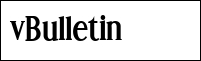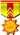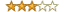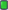ExetoolsGMP function
 Register Forum Rules FAQ Calendar Mark Forums Read

 Notices https://forum.exetools.com This is the ONLY ONE domain that we use. Follow @exetools on Twitter and send me a message, I will choose whether to send the invitation code. Any password problems please mailto: 883600(at)qq(dot)com

#1GitOld GitJoin Date: Mar 2002 Location: Torino Posts: 1,102 Rept. Given: 221 Rept. Rcvd 265 Times in 157 Posts Thanks Given: 98 Thanks Rcvd at 218 Times in 124 PostsGMP function

Anybody familiar enough with GMP (Gnu MP big number library) to identify this function?. None of the gmp sigs I have tried recognise any of the library functions but I am certain it is GMP or a derivative. I may have misidentified the 16byte struct type that the parameter points to.

Code:
```.text:10001000 ; void __cdecl SUB01(mpf_ptr x)
.text:10001000 SUB01           proc near
.text:10001000
.text:10001000 x               = dword ptr  4
.text:10001000
.text:10001000                 mov     ecx, [esp+x]
.text:10001004                 mov     edx, [ecx+mpf_struct._mp_size]
.text:10001007                 mov     eax, [ecx+mpf_struct._mp_prec]
.text:10001009                 push    esi
.text:1000100A                 mov     esi, edx        ; esi = x->_mp_size
.text:1000100C                 ror     esi, 5          ; esi = ROR(x->_mp_size, 5)
.text:1000100F                 sub     eax, esi        ; eax = x->_mp_prec - ROR(x->_mp_size, 5)
.text:10001011                 mov     esi, [ecx+mpf_struct._mp_exp] ; esi = x->_mp_exp
.text:10001014                 push    edi
.text:10001015                 mov     edi, esi        ; edi = x->_mp_exp
.text:10001017                 ror     edi, 0Fh        ; edi = ROR(x->_mp_exp, 15)
.text:1000101A                 xor     edi, edx        ; edi = ROR(x->_mp_exp, 15) ^ x->_mp_size
.text:1000101C                 mov     edx, [ecx+mpf_struct._mp_d] ; edx = x->_mp_d
.text:1000101F                 add     esi, edx        ; esi = x->_mp_exp + x->_mp_d
.text:10001021                 add     edx, eax        ; edx = x->_mp_d + x->_mp_prec - ROR(x->_mp_size, 5)
.text:10001023                 mov     [ecx+mpf_struct._mp_exp], edx ; x->_mp_exp = x->_mp_d + x->_mp_prec - ROR(x->_mp_size, 5)
.text:10001026                 mov     edx, edi        ; edx = ROR(x->_mp_exp, 15) ^ x->_mp_size
.text:10001028                 mov     [ecx+mpf_struct._mp_prec], edi ; x->_mp_prec = ROR(x->_mp_exp, 15) ^ x->_mp_size
.text:1000102A                 add     eax, edx        ; eax = x->_mp_prec - ROR(x->_mp_size, 5) + ROR(x->_mp_exp, 15) ^ x->_mp_size
.text:1000102C                 pop     edi
.text:1000102D                 mov     [ecx+mpf_struct._mp_size], esi ; x->_mp_size = x->_mp_exp + x->_mp_d
.text:10001030                 mov     [ecx+mpf_struct._mp_d], eax ; x->_mp_d = x->_mp_prec - ROR(x->_mp_size, 5) + ROR(x->_mp_exp, 15) ^ x->_mp_size
.text:10001033                 pop     esi
.text:10001034                 retn
.text:10001034 SUB01           endp
.text:10001034

void __cdecl SUB01(mpf_ptr x)
{
x->_mp_exp = x->_mp_d + x->_mp_prec - ROR(x->_mp_size, 5)
x->_mp_prec = ROR(x->_mp_exp, 15) ^ x->_mp_size
x->_mp_size = x->_mp_exp + x->_mp_d
x->_mp_d = x->_mp_prec - ROR(x->_mp_size, 5) + ROR(x->_mp_exp, 15) ^ x->_mp_size    // can simplify
return
}```
#2
 KerlingenVIPJoin Date: Feb 2011 Posts: 310 Rept. Given: 0 Rept. Rcvd 276 Times in 98 Posts Thanks Given: 0 Thanks Rcvd at 270 Times in 85 PostsI didn't know GMP before, but I tried to find you code in GMP4 and GMP5. I tried finding the "ror" part, since I think it's very unusual.

The source doesn't contain any matching "ror" in the *.asm files.
The source doesn't contain any matching pseudo-C "ror" like "(x>>n | x<<32-n)" in any file.
The compiled GMP4 and GMP5 libraries don't contain any "ror" instruction.

Maybe it's a different GMP version or different compiler settings where used.

I think the function doesn't modify a "mpf_ptr" structure, the arithmetic operations don't really fit the structure.
#3GitOld GitJoin Date: Mar 2002 Location: Torino Posts: 1,102 Rept. Given: 221 Rept. Rcvd 265 Times in 157 Posts Thanks Given: 98 Thanks Rcvd at 218 Times in 124 PostsNo, having looked further, I believe the struct is based on the 12 byte mpz_struct type with 4 bytes for another field. I also think it may be a custom random generator. The function that calls it certainly looks like a random seeding procedure. It's also possible that the ror has arisen from compiler optimisation, but of what I don't know.

Thanks for taking the time to look.

Git
#4
 KerlingenVIPJoin Date: Feb 2011 Posts: 310 Rept. Given: 0 Rept. Rcvd 276 Times in 98 Posts Thanks Given: 0 Thanks Rcvd at 270 Times in 85 PostsCode:
```_C = D + A - ROR(B, 5)
_A = ROR(C, 15) xor B
_B = C + D
_D = A - ROR(B, 5) + ROR(C, 15) xor B
A = _A
B = _B
C = _C
D = _D```
This looks like the inner loop of some hash or crypto function.

A "mpz_struct" doesn't fit either. Both structures contain a pointer and XORing pointers isn't the best idea, even if adding or subtracting is sometimes used with pointers. Do you have a link to the code you're analysing? Just this one code fragment doesn't show much.
#5GitOld GitJoin Date: Mar 2002 Location: Torino Posts: 1,102 Rept. Given: 221 Rept. Rcvd 265 Times in 157 Posts Thanks Given: 98 Thanks Rcvd at 218 Times in 124 PostsYes, it is some sort of hash function used to return a randomish sequence of numbers. The struct is something like :

struct hash_ctx
{
ULONG salt;
ULONG a0;
ULONG a1;
ULONG a2;
}

An initialising function sets salt to an obscure 32 bit constant and a0=a1=a2 to a second parameter and then calls the hash function. The hash function is then called successively returning the value in eax which is the same as field ctx->a2.

Git

 Thread ToolsShow Printable VersionEmail this Page Display ModesLinear ModeSwitch to Hybrid ModeSwitch to Threaded ModePosting Rules You may not post new threads You may not post replies You may not post attachments You may not edit your posts BB code is On Smilies are On [IMG] code is Off HTML code is Off Forum RulesSimilar Threads Thread Thread Starter Forum Replies Last Post Git General Discussion 6 09-24-2014 01:58 Git General Discussion 4 09-07-2005 19:35 Peter[Pan] General Discussion 8 08-31-2004 20:37

All times are GMT +8. The time now is 09:20.

 Aaron's homepage - Top